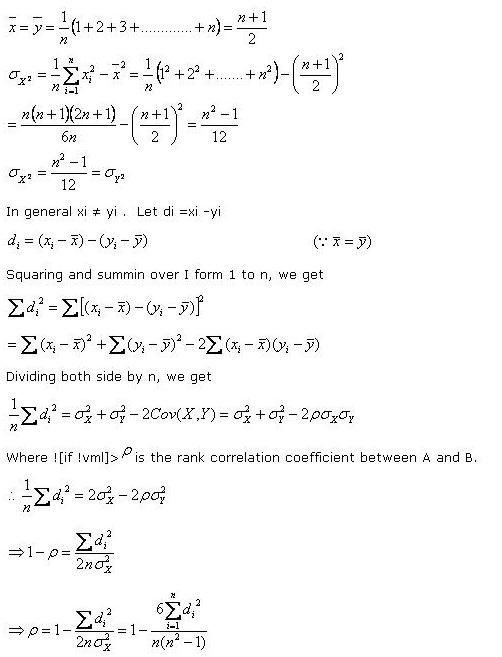# Statistics Assignment Help With Rank Correlation

## Spearman’s Rank Correlation CoefficientThe spearman’s rank coefficient of correlation was developed by Charles Edward Spearman.

### What is Spearman correlation coefficient?

Let us suppose that a group of n individuals is arranged in order of merit or proficiency in possession of two characteristics A and B. these ranks in two characteristics will, in general, be different. For example, if we consider the relation between intelligence and beauty, it is not necessary that a beautiful individual is intelligent also. Let (xi , yi ); i=1,2,………,n be the ranks of the ith individual in two characteristics A and B respectively. Pearsonian coefficient of correlation between the ranks xi’s and yi ‘s is called the Spearman rank correlation coefficient between A and B for that group of individuals.

### The formula for correlation of rank coefficient is given as:

rk = 1 – [6 ∑D2 / N3 – N]

Where D = R1 – R2, between the paired items in the two rank series.

The value of rank correlation coefficient tells us about the degree of agreement between the 2 ranks.

#### See derivation for the rank coefficient of correlation by spearman below:

Assuming that no two individuals are bracketed equal in either classification, each of the variables X and Y takes the values 1,2,………..,n

Hence

###Which is the spearman's formula for the rank correlation coefficient.

### Properties of Spearman’s Rank correlation Coefficient

-1 ≤ rk ≤ +1

Rank Correlation Coefficient Example

Calculate the Rank Correlation Coefficient in each of the following cases:

XR1YR2
10151
20262
30373

To calculate the rank correlation coefficient, first we will determine the value of D = R1 – R2 in each of the entries:

XR1YR2DD2
1015100
2026200
3037300

Then the Spearman’s rank correlation coefficient is calculated using the formula as:

rk = 1 – [6 ∑D2 / N3 – N]

= 1- 6(0)

= +1

Thus the value of rank correlation coefficient equal to +1 implies that there is complete agreement in the order of ranks and the ranks are in the same direction.

Let us calculate the rank correlation coefficient in another example:

XR1YR2
10173
20262
30351

To calculate the rank correlation coefficient, first we will determine the value of D = R1 – R2 in each of the entries:

XR1YR2DD2
10173-24
2026200
30351+24

Then the Spearman’s rank correlation coefficient is calculated using the formula as:

rk = 1 – [6 ∑D2 / N3 – N]

= 1 – (6*8)/9-3

= -1

Thus the value of rank correlation coefficient equal to -1 implies that there is complete agreement in the order of ranks and the ranks are in opposite direction